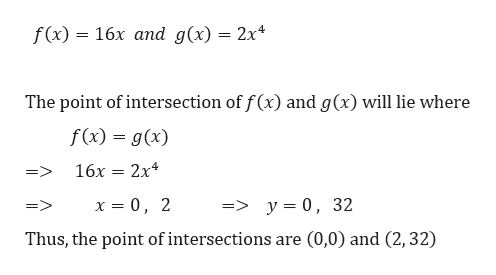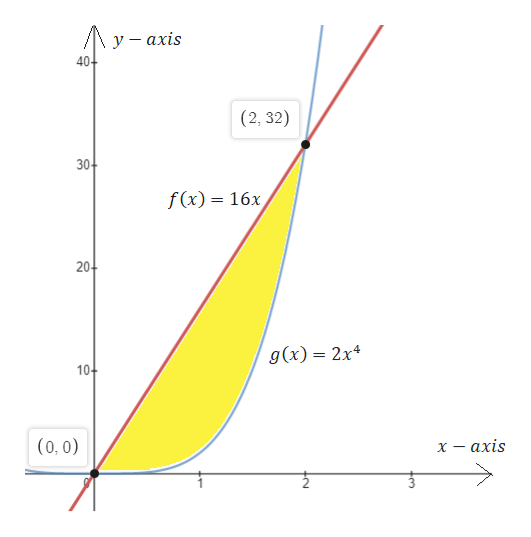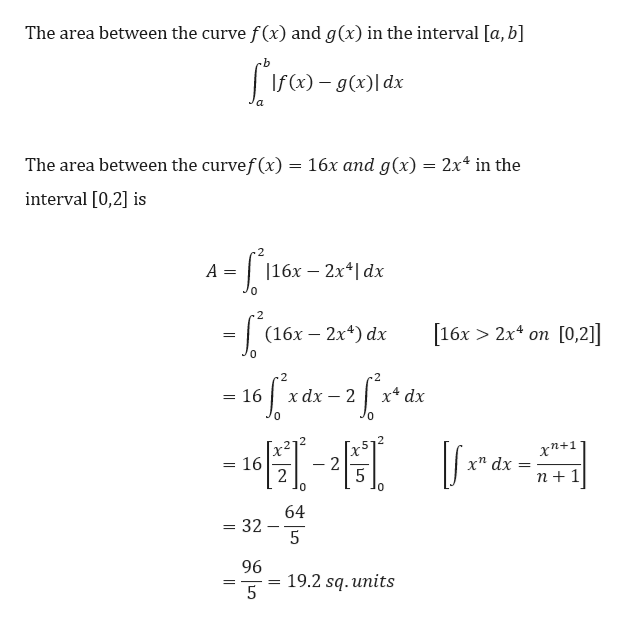# Find the area enclosed by the curves f(x) = 16x and g(x) =2x4

Question
4 views

Find the area enclosed by the curves f(x) = 16x and g(x) =2x4

check_circle

Step 1

We need to find the area enclosed by the curveshelp_outlineImage Transcriptionclosef(x) = 16x and g(x) = 2x* The point of intersection of f (x) and g(x) will lie where f(x) = g(x) 16x = 2x* => x = 0, => y = 0, 32 => Thus, the point of intersections are (0,0) and (2, 32) fullscreen
Step 2

The region enclosed by f(x) and g(x) can be easily shown in the plot belowhelp_outlineImage Transcriptionclose/Ay- αxis у—ахis 40+ (2, 32) 30- f(x) = 16x 20- g(x) = 2x+ 10- (0, 0) axis fullscreen
Step 3

We know th...help_outlineImage TranscriptioncloseThe area between the curve f(x) and g(x) in the interval [a, b] | If(x) – g(x)| dx The area between the curvef(x) = 16x and g(x) = 2x* in the interval [0,2] is 116х — 2x4] dx A = [16х > 2х4 оп [О,2] (16х — 2х4) dx Je 16[* 2 х4 dx хаx — 2 хп+1 = 16 - 2 х^ dx n +1 64 - 32 5 96 19.2 sq. units fullscreen

### Want to see the full answer?

See Solution

#### Want to see this answer and more?

Solutions are written by subject experts who are available 24/7. Questions are typically answered within 1 hour.*

See Solution
*Response times may vary by subject and question.
Tagged in

### Integration